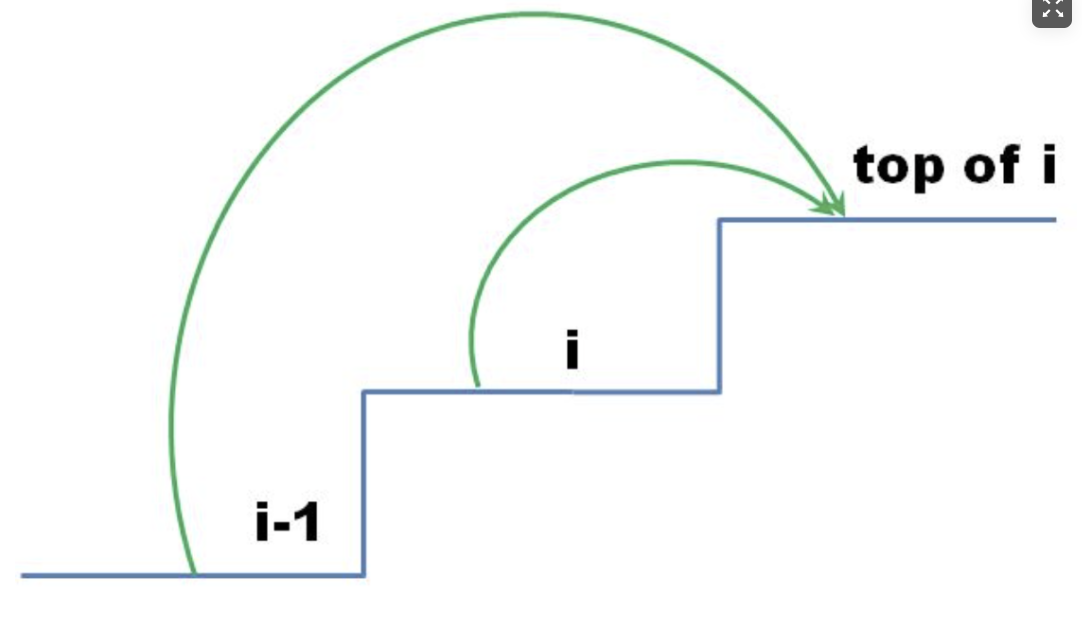# 算法攻关 - 爬楼梯最小花费 _0076# 一、题目描述

示例 2：

cost 的长度范围是 [2, 1000]。

cost[i] 将会是一个整型数据，范围为 [0, 999] 。

# 二、思考dp[i]的定义：第 i 个台阶所花费的最少体力为 dp[i]。（注意这里认为是第一步一定是要花费）

int[] dp(cost.size()); dp = cost; dp = cost;

1. 确定遍历顺序

# 三、代码实现

class Solution {    public int minCostClimbingStairs(int[] cost) {        //初始化状态数组        int[] dp = new int[cost.length];        //处理0和1        dp = cost;        dp = cost;        //演变递推公式        for (int i = 2; i < cost.length; i++) {            dp[i] = Math.min(dp[i - 1], dp[i - 2]) + cost[i];        }        // 注意最后一步可以理解为不用花费，所以取倒数第一步，第二步的最少值        return Math.min(dp[cost.length - 1], dp[cost.length - 2]);    }}

class Solution {    public int minCostClimbingStairs(int[] cost) {        //直接利用数组来代表有状态的数组        for (int i = 2; i < cost.length; i++) {            cost[i] = Math.min(cost[i - 2], cost[i - 1]) + cost[i];        }        return Math.min(cost[cost.length - 2], cost[cost.length - 1]);    }}

# 四、小结## 评论